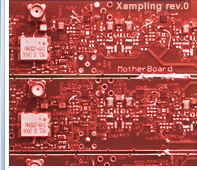About Me Publications Graduate Students Courses Software & Hardware Xampling SAMPL Lab Press Watch Events LinksHome Contact MeXampling Sequences of Innovation Multipath Medium Identification Using Efficient Sampling Schemes Kfir Gedalyahu and Yonina C. Eldar Introduction The problem of time delay estimation arises in many applications in which a multipath medium has to be identified using pulses transmitted through the medium. Various approaches have been proposed in the literature to identify the delays introduced by the multipath environment, however these methods require high sampling rates in order to achieve reasonable time resolution. In this work we present a new method, allowing the recovery of the medium parameters from sub-Nyquist samples. Our approach can identify a medium comprised of L propagation paths, by sampling its output at a rate of 2L/T, irrespective of the pulse bandwidth. Signal Model The medium is assumed to be probed with a known pulse at a constant rate of 1/T. The received signal from the medium is comprised of L pulses per period, corresponding to L propagation paths. The delays in each period are assumed to be constant, where the reflection coefficients of the pulses can vary from period to period. There is no constraint on the pulses support, and they can have infinite time support. Mathematically such a model is defined aswhere ak[n] and tk are the reflection coefficient and delay of the kth propagation path, and g(t) is the pulse shape. An example of a received signal from a medium with L=2 propagation paths is given below:Another possible application for such signal model is high-resolution radar. Xampling System The proposed method is comprised of three main stages: 1. Sampling stage: The input signal is sampled using p parallel sampling channels. Each channel consists of a sampling filter si(t), followed by a uniform sampler operating at a rate of 1/T. The number of sampling channels has to be greater or equal 2L, leading to an average sampling rate of 2L/T.  Examples of filters selections: A set of 2L ideal complex band-pass ﬁlters, each one covering a different frequency band of width 1/T. Low pass filter with a width of 2L/T, followed by a uniform sampler operating at a rate of 2L/T. In this case the parallel sampling sequences are obtained by a serial to parallel  converter, as we will show next. 2. Equalization stage: The sampling sequences at the output of the sampling stage are filtered using a properly designed digital filter-bank  W-1(ejwT) (see  for details). 3. Reconstruction stage: After the equalization stage the delays are recovered from the samples at the output of the digital filter-bank the using the well known ESPRIT algorithm. Then, the reflection coefficients are recovered using additional digital filter-bank. The full sampling and reconstruction scheme is given belowOne special case of the proposed scheme, is when only one physical sampling channel is used. In this case a low-pass filter is used as a sampling kernel, followed by a uniform sampler at a rate of 2L/T. The parallel sampling sequences are obtained using a serial-to-parallel device. Moreover, it can be shown that in this case the first digital correction filter bank, can be decomposed into three stages: two stages of parallel one-dimensional filters Ψi(ejwT) and Φi(ejwT) and FFT operation.We now provide an example demonstrating the importance of the sampling filter. We consider the case where the input signal is comprised of L=2 Diracs per period. In the following figure, we show the output of one of the sampling channels. This example demonstrates the need for the sampling kernel when sampling short-length pulses at a low sampling rate. The sampling kernels have the effect of smoothing the short pulses (Diracs in this example). Consequently, even when the sampling rate is low, the samples contain information about the signal. In contrast, if we were to sample the input signal directly at a low rate, then we would often obtain only zero samples which contain no information about the signal.Channel Identification Example An example for identification of a multipath channel is depicted below. The channel consists of L=4 propagation paths. The medium is assumed to be probed with Diracs at a rate of 1/T. The recovery of the medium parameters is performed from noisy samples with SNR of 10dB taken at a rate of 8/T. The original and estimated delays and average energy of each propagation path are shown in the figure. Our method provides good estimation of the channel parameters. In contrast, in this setting sampling at the Nyquist rate is inapplicable since the pulses are not band-limited.Next, we explore the performance of our method in the presence of noise, for the above channel setting. The MSE of the delays estimation is plotted as a function of the samples SNR. It can be seen that for SNR above 10dB, our method achieves the Cramer-Rao bound (CRB).Reference K. Gedalyahu and Y. C. Eldar, "Time Delay Estimation from Low Rate Samples: A Union of Subspaces Approach", IEEE Trans. Signal Processing, vol. 58, no. 6, pp. 3017–3031, June 2010. Software Download Installation: Unzip all files to a directory of your choice. Usage: Run LRTDE.m. Instructions on the GUI operation can be found in LRTDE_GUI.docx. Installation: Unzip all files to a directory of your choice. Usage: Use AnalogSampling.m to perform sampling of a multipath channel ouput. Use ChanRecover.m to perform recovery of the channel's parameters. For more information on the functions usage see LRTDE_Package.docx. XamplingThe Big PictureWideband Communication and Cognitive RadioMultipath Channel IdentificationPulse Streams with Medical ApplicationSub-Nyquist Ultrasound ImagingMultichannel Pulse StreamsSuper-Resolution RadarCopyright © 2010 Professor Yonina Eldar. All Rights Reserved.